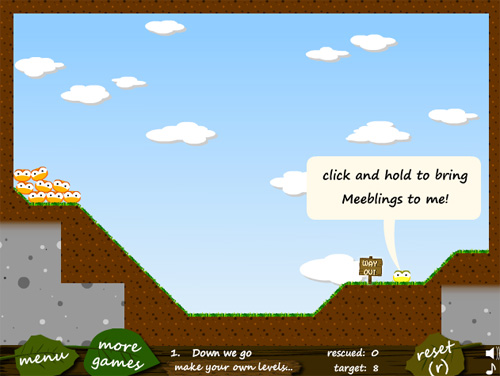# Prototype of a Flash game like Meeblings

You should all known Meeblings and the sequel, Meeblings 2.You have to help the Meeblings (cute creatures I’d love to burn alive) reaching the exit using some special abilities.

One of such abilities attracts Meeblings when you click and hold the mouse on a special Meebling.

In the prototype you are about to see, derived from the basic HelloWorld Box2D example, there are 10 randomly placed balls with different masses.

When you click and hold anywhere on the stage, every ball in a 4 meters radius (read this post and this post too if you don’t know how to convert meters to pixels) will be attracted towards the mouse pointer.

The more the distance, the stronger the attraction.

```package {
import flash.display.Sprite;
import flash.events.Event;
import Box2D.Dynamics.*;
import Box2D.Collision.*;
import Box2D.Collision.Shapes.*;
import Box2D.Common.Math.*;
import flash.events.MouseEvent;
public class HelloWorld extends Sprite {
var body:b2Body;
var magnet:Boolean=false;
public function HelloWorld() {
var worldAABB:b2AABB = new b2AABB();
worldAABB.lowerBound.Set(-100.0, -100.0);
worldAABB.upperBound.Set(100.0, 100.0);
var gravity:b2Vec2=new b2Vec2(0.0,10.0);
var doSleep:Boolean=true;
m_world=new b2World(worldAABB,gravity,doSleep);
var m_sprite:Sprite;
m_sprite = new Sprite();
var dbgDraw:b2DebugDraw = new b2DebugDraw();
var dbgSprite:Sprite = new Sprite();
dbgDraw.m_sprite=m_sprite;
dbgDraw.m_drawScale=30;
dbgDraw.m_alpha=1;
dbgDraw.m_fillAlpha=0.5;
dbgDraw.m_lineThickness=1;
dbgDraw.m_drawFlags=b2DebugDraw.e_shapeBit;
m_world.SetDebugDraw(dbgDraw);
var bodyDef:b2BodyDef;
var boxDef:b2PolygonDef;
var circleDef:b2CircleDef;
bodyDef = new b2BodyDef();
bodyDef.position.Set(8, 14);
boxDef = new b2PolygonDef();
boxDef.SetAsBox(80, 1);
boxDef.friction=0.3;
boxDef.density=0;
body=m_world.CreateBody(bodyDef);
body.CreateShape(boxDef);
body.SetMassFromShapes();
for (var i:int = 1; i <= 10; i++) {
bodyDef = new b2BodyDef();
bodyDef.position.x=Math.random()*12+2;
bodyDef.position.y=Math.random()*5;
circleDef = new b2CircleDef();
circleDef.density=1.0;
circleDef.friction=0.5;
circleDef.restitution=0.2;
body=m_world.CreateBody(bodyDef);
body.CreateShape(circleDef);
body.SetMassFromShapes();
}
}
public function createMouse(evt:MouseEvent):void {
magnet=true;
}
public function destroyMouse(evt:MouseEvent):void {
magnet=false;
}
public function Update(e:Event):void {
var dist_x;
var dist_y;
var distance;
m_world.Step(m_timeStep, m_iterations);
if (magnet) {
for (var bb:b2Body = m_world.m_bodyList; bb; bb = bb.m_next) {
dist_x=mouseX/30-bb.GetPosition().x;
dist_y=mouseY/30-bb.GetPosition().y;
distance = dist_x*dist_x+dist_y*dist_y;
if (distance<16) {
bb.ApplyImpulse(new b2Vec2(dist_x/20, dist_y/5),bb.GetWorldCenter());
}
}
}
}
public var m_world:b2World;
public var m_iterations:int=10;
public var m_timeStep:Number=1.0/30.0;
}
}```

The only new, interesting line is line 78 where I apply an impulse as strong as the distance between the ball and the mouse, once I checked the player is holding mouse button and the distance from the ball and the mouse is less than 4 meters.

Look how I divide `dist_x` and `dist_y` by different numbers to balance gameplay.

This is the result:

214 GAME PROTOTYPES EXPLAINED WITH SOURCE CODE
// 1+2=3
// 10000000
// 2 Cars
// 2048
// Avoider
// Ballz
// Block it
// Blockage
// Bloons
// Boids
// Bombuzal
// Breakout
// Bricks
// Columns
// CubesOut
// Dots
// DROP'd
// Dudeski
// Eskiv
// Filler
// Fling
// Globe
// HookPod
// Hundreds
// InkTd
// Iromeku
// Lumines
// Magick
// MagOrMin
// Maze
// Memdot
// Nano War
// Nodes
// o:anquan
// Ononmin
// Pacco
// Phyballs
// Platform
// Poker
// Pool
// Poux
// Pudi
// qomp
// Racing
// Renju
// SameGame
// Security
// Sling
// Slingy
// Sokoban
// Splitter
// Sproing
// Stack
// Stringy
// Sudoku
// Tetris
// Threes
// Toony
// Turn
// TwinSpin
// vvvvvv
// Wordle
// Worms
// Yanga
// Zhed
// zNumbers